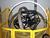## RPG array questionTags:
RPG
RPG arrays
Hello all, I have an Array (@K1) that I do not quite understand and was looking for clarity. The array (@K1) sets a counter var (\$I) at 1, then a LOKUP@K1, \$I is executed using \$K1 as the key, if found it sets on *IN90. What I do not understand is that right after that, if *IN90 is off, it uses *BLANKS to do the same LOKUP and sets on *IN90 if found. Here are my questions: Is this an old programming trick to find some type of record even though it uses *BLANKS as the key? If *IN90 is on (1) it adds the \$K1 to the arrays, correct? Here’s the code:
```Z-ADD1 \$I 40
\$K1 LOKUP@K1,\$I 90
*IN90 IFEQ '0'
*BLANKS LOKUP@K1,\$I 90
END

*IN90 IFEQ '1'
MOVEL\$K1 @K1,\$I
SUB QPGPA @A1,\$I
END```
Any help would be greatly appreciated. Thanks! Jeff
Related Questions

Thanks. We'll let you know when a new response is added.

## Discuss This Question: 3 Replies

Thanks. We'll let you know when a new response is added.
•report
•Perhaps this will help
```Z-ADD1 		\$I 	40   <- \$I = 1
\$K1 LOKUP@K1,\$I 90	     <- search in array @K1 for value\$K1 put location into \$I
<- if the 90 is in positions (58-59): Instructs the program to find the entry equal to the search                                 argument. The first equal entry found sets the indicator assigned to equal on.

*IN90 IFEQ '0'		     <- if the value was not found in the array
*BLANKS LOKUP@K1,\$I 90	     <- find the first blank in the table .. put locatin into \$I
END

*IN90 IFEQ '1'		     <- if either the first lokup found the value and put the location in \$I
<- or the second lokup found a blank and put that location in \$I
MOVEL\$K1 @K1,\$I		     <- put the current value \$K1 into aray @K1 at location \$I
<- this is redundant if the first lokup was successful
SUB QPGPA @A1,\$I             <- Subtract the value QPGPA from the value in Array @A1 in location \$I
END```
report
• This is what I needed to validate... thanks for your help

*BLANKS LOKUP@K1,\$I 90

^- find the first blank in the table .. put location into \$I

report